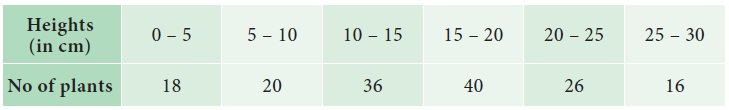Home | | Statistics 11th std | Percentiles

# Percentiles

The percentile values divide the frequency distribution into 100 parts each containing 1 percent of the cases.

Percentiles

The percentile values divide the frequency distribution into 100 parts each containing 1 percent of the cases. It is clear from the definition of quartiles, deciles and percentiles

Relationship

P25 = Q1

P50 = Median = Q2

P75 = 3rd quartile = Q3

### Example 5.35

The following is the monthly income (in 1000) of 8 persons working in a factory. Find P30 income value

10,14, 36, 25, 15, 21, 29, 17

### Solutions:

Arrange the data in an ascending order.

n = 8

10,14,15,17,21,25,29,36### Example 5.36

Calculate P61 for the following data relating to the height of the plants in a garden### Solution:Tags : Definition, Solved Example Problems , 11th Statistics : Chapter 5 : Measures of Central Tendency
Study Material, Lecturing Notes, Assignment, Reference, Wiki description explanation, brief detail
11th Statistics : Chapter 5 : Measures of Central Tendency : Percentiles | Definition, Solved Example Problems